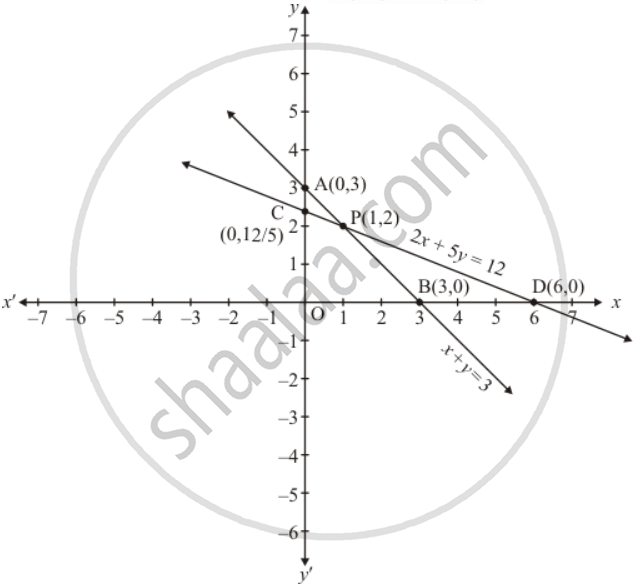Share

# Solve the Following Systems of Equations Graphically: X − 2y = 5 2x + 3y = 10 - CBSE Class 10 - Mathematics

ConceptGraphical Method of Solution of a Pair of Linear Equations

#### Question

Solve the following systems of equations graphically:

x − 2y = 5

2x + 5y = 12

#### Solution

The given equations are:

x + y = 3 ....(1)

2x + 5y = 12 ....(2)

Putting x = 0 in equation 1 we get:

=> 0 + y = 3

=> y = 3

x = 0, y = 3

Putting y = 0  in equation 1 we get

=> x + 0 = 3

=> x = 3

x = 3 , y = 0

Use the following table to draw the graph.

 x 0 3 y 3 0

Draw the graph by plotting the two points A(0,3) and B(3,0) from tableGraph of the equation (2)

=> 2x + 5y = 12

Putting x = 0 in equation (2), we get

=> 2 xx 0 + 5y = 12

=> 5y = 12

=> y = 12/5

x = 0, y = 12/5

Putting y = 0 in  equation (2) we get

=> 2x + 5 xx 0 = 12

=> 2x = 12

=> x = 6

x = 6, y = 0`

Use the following table to draw the graph.

 x 0 6 y 12/5 0

Draw the graph by plotting the two points C(0, 12/5), D(6,0) from the table.

The two line intersect at point P(1,2)

Hence x = 1 and y = 2 is the solution

Is there an error in this question or solution?

#### APPEARS IN

Solution Solve the Following Systems of Equations Graphically: X − 2y = 5 2x + 3y = 10 Concept: Graphical Method of Solution of a Pair of Linear Equations.
S